#### Graph matching (a.k.a. network alignment)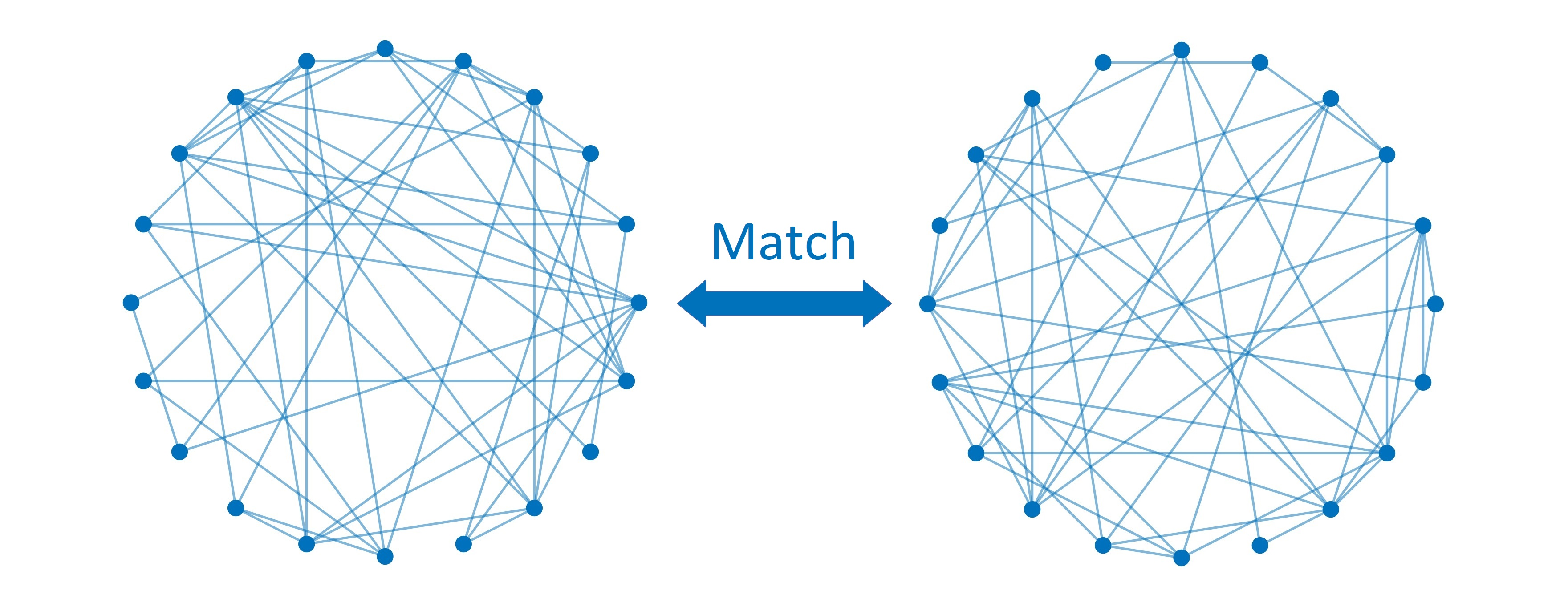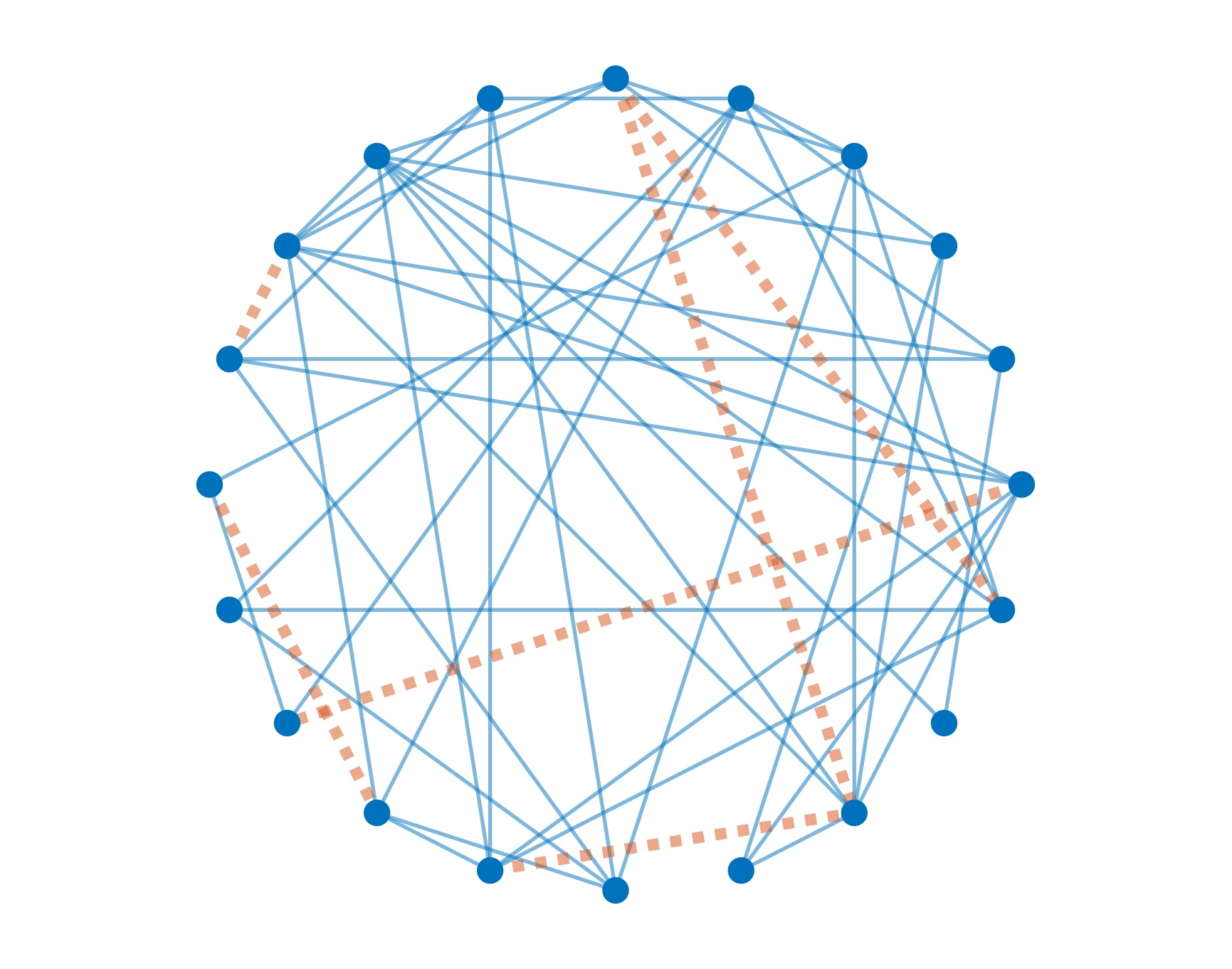The problem of graph matching consists in matching the vertices of two unlabeled, edge-correlated graphs so that their edges are maximally aligned. The above two graphs on the left are edge-correlated but their vertices are unmatched, so they appear to be quite different. On the right-hand side, the two graphs are optimally aligned so that there are only a few misaligned edges (dotted red lines). In the following papers, we developed a full-rank spectral method for graph matching, which is implemented here.

#### Mixture models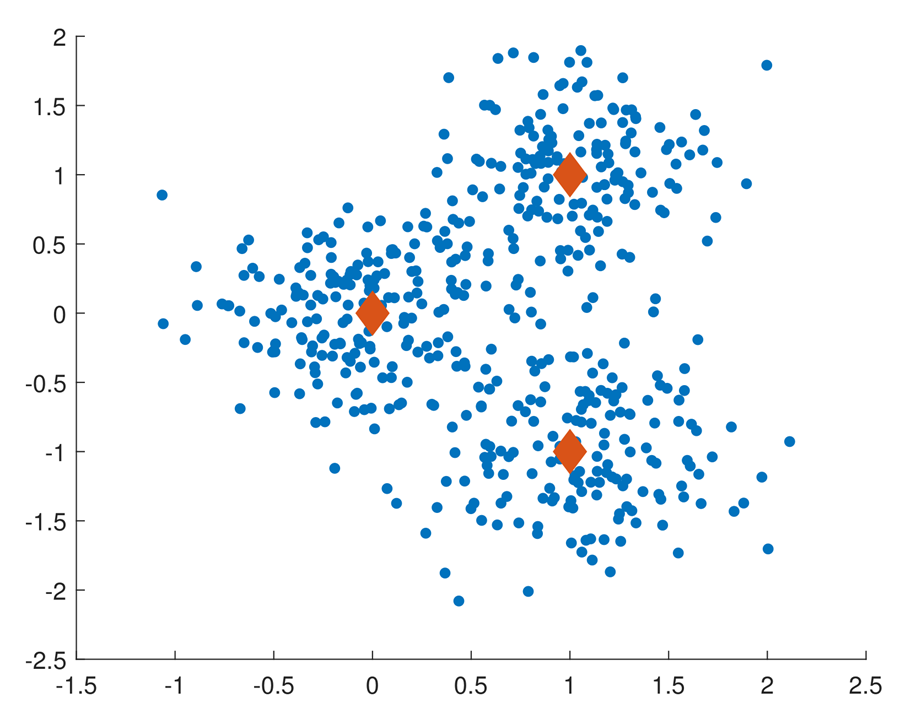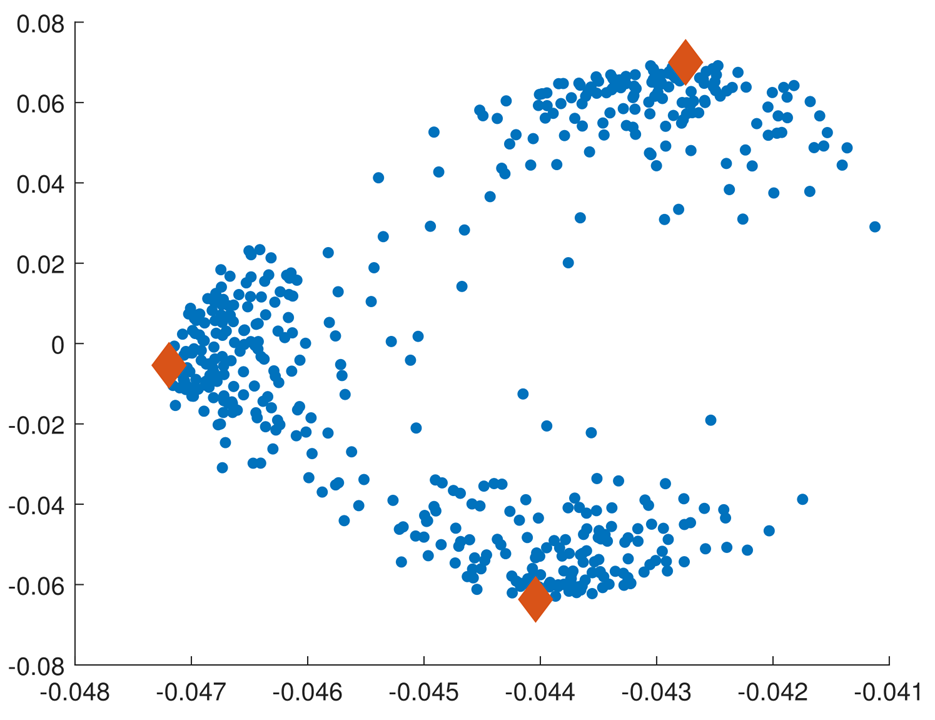Mixture models are used to represent data from heterogeneous populations. This research in progress aims to develop algorithmic and analytic tools for both mixtures in the Euclidean space and mixtures of permutations.

#### Ranking from pairwise comparisons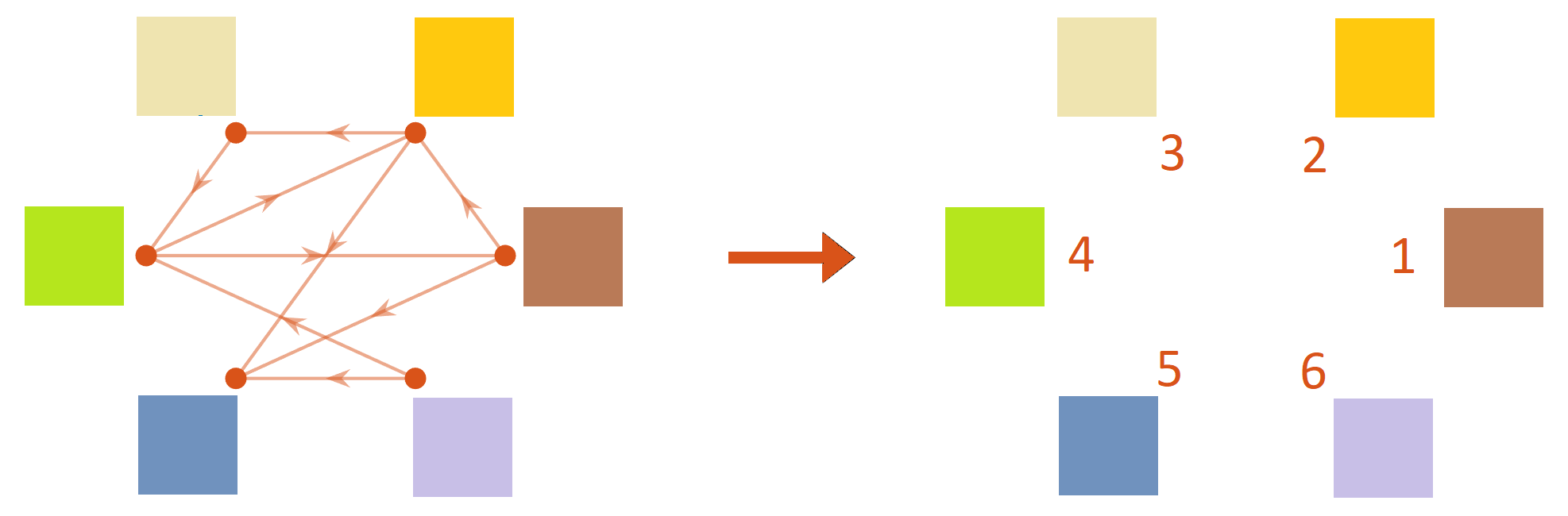Ranking from pairwise comparisons, as the name suggests, is the task of aggregating a set of comparisons between pairs of items to produce a ranking of the items. I have particularly worked on a class of permutation-based models with co-authors in the following works.

#### Other problems with latent permutations and shape constraints

Some of my other research also concerns statistical estimation with latent combinatorial structure and/or shape constraints.